# Some Questions About Vela Compiler Report

Hi,

I recently trained two model, and used vela compiler to estimate their performance.

There are their model architecture :

(I changed the right model's middle layer from "Standard Convolution(Conv2D_16x3x3x16(NHWC))" to "Depthwise Seperable Convolution(DepthwiseConv2D_1x3x3x16(NHWC)+Conv2D_16x1x1x16(NHWC))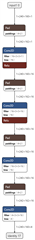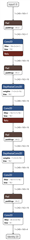I compiled them with the same system config and memory mode.

(I selectd accelerator ethos-u55-128 and add --optimise Performance command)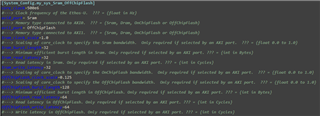(System Config)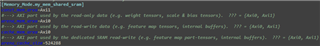(Memory Mode)

Finally,there are their vela report :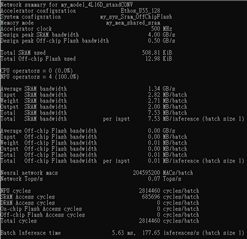(Model with all Standard Conv)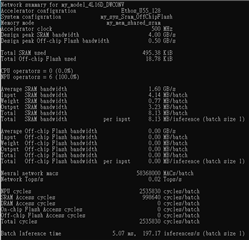(Model with Depthwise Seperable Conv)

I compared them and had some question :

1. In "Total SRAM Bandwidth", why the model with depthwise conv is bigger than the model with all standard conv ?

Is this means depthwise conv will transfer the feature map between external SRAM and NPU internal SRAM more frequently?

2. In "Neural network macs", the numbers of depthwise conv is 3.5 times smaller than the standard conv one, but only reduce the "NPU cycles" from 2814460 to 2535830 .

It seems that using depthwise conv to improve the model's inference time doesn't get very good effect.

I read the Ethos-U55 NPU Technical Reference Manual(https://developer.arm.com/documentation/102420/0200/?lang=en), and found that the table in topic 4.8 "Operator and Performance"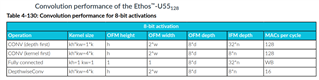In this table, Depthwise Conv only use 16 MAC per cycle.

So I think the reason of this question is that DepthwiseConv has lower MAC utilization than standard Conv. Are there other reasons ?

1. Yes, it does. This is because the the block-based architecture of the Ethos-U hardware does a better job of "caching" the IFM on the 'Standard Conv' network. Also, the 'Depthwise Conv' network contains two operators and so requires two OFMs to be written.
2. Yes, you are correct, the Depthwise Conv operator will only use 16 out of the 128 MACs, per cycle. However, there are also other factors which need to be taken into account. These are the kernel size and IFM depth. The following theoretical performance calculations will hopefully explain this more clearly (all numbers are taken from the Operator Performance table):
• 'Standard Conv' network
• CONV2D
• The IFM depth is 16, therefore the compiler will select CONV 'kernel first' as this processes the IFM depth in multiples of 8 (as opposed to 32 with 'depth first') => 100% utilisation
• The kernel is 3x3 = 9 which is processed in multiples of 4. So, 9/12 => 75% utilisation
• MAC utilisation= 100% * 75% = 75%
• Operator cycles = 88,473,600 MACs at 128 MACs per cycle with 75% utilisation= 921,600 cycles
• Network cycles = 921,600 cycles
• 'Depthwise Conv' network
• DEPTHWISE_CONV
• The operator only uses 16 out of the 128 MACs => 12.5% utilisation
• The kernel is 3x3 = 9 which is process in multiples of 4 => 75% utilisation
• MAC utilisation= 12.5% * 75% = 9.375%
• Operator cycles = 5,529,600 MACs at 128 MACs per cycle with 9.375% utilisation= 460,800 cycles
• CONV2D
• The IFM depth is 16, therefore the compiler will select CONV 'kernel first' as this processes the IFM depth in multiples of 8 (as opposed to 32 with 'depth first') => 100% utilisation
• The kernel is 1x1 = 1 which is process in multiples of 4. So, 1/4 => 25% utilisation
• MAC utilisation= 100% * 25% = 25%
• Operator cycles = 9,830,400 MACs at 128 MACs per cycle with 25% utilisation= 307,200 cycles
• Network cycles = 460,800 + 307,200 = 768,000 cycles

Whilst these numbers roughly match those of Vela's performance estimator the best way to determine the real performance would be to run on an FPGA or even the FVP. The performance results of the FVP show that the 2 networks are almost identical in performance:

• 'Standard Conv' network = 143,146 cycles
• 'Depthwise Conv' network = 143,025 cycles